Function Repository Resource:

# FrobeniusPiecewiseDSolve

Obtain an approximate solution to an ODE using the piecewise Frobenius method

Contributed by: Rauan Kaldybayev
 ResourceFunction["FrobeniusPiecewiseDSolve"][eqn,u,x,x0,{xmin,xmax}] computes an approximate solution to a differential equation for the function u, with independent variable x, in the interval xmin≤x≤xmax using the piecewise Frobenius method. ResourceFunction["FrobeniusPiecewiseDSolve"][x,x0,{xmin,xmax},formula] quickly computes an approximate piecewise Frobenius solution using the algebraic formula supplied by the resource function FrobeniusPiecewiseDSolveFormula.

## Details and Options

The options for ResourceFunction["FrobeniusPiecewiseDSolve"] are:
 Tolerance 10-3 the allowed error margin (as defined below) of the approximate solutions "FrobeniusOrder" 28 the order of each of the Frobenius series constituting the piecewise solutions
ResourceFunction["FrobeniusPiecewiseDSolve"] computes piecewise Frobenius solutions - that is, in each of the piecewise subintervals, the approximate solution is given by a Frobenius series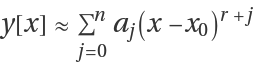.
"FrobeniusOrder" is the value of n in each of the Frobenius series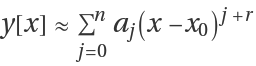. The higher "FrobeniusOrder" is, the more accurate is each of the series solutions, and the less of these solutions are needed to cover the domain of interest. However, making "FrobeniusOrder" too large results in underflow and subsequent issues (see Possible Issues).
Decreasing Tolerance means that more subintervals have to be used to maintain the desired accuracy, which in turn increases computation time. However, thanks to the properties of series solutions, the dependency of computation time on Tolerance is pretty weak (see Options).
ResourceFunction["FrobeniusPiecewiseDSolve"] can solve linear homogeneous ODEs with polynomial coefficients.
The output is of the form {soln1, soln2, solnZ}, where Z is the order of the ODE and the solni are linearly independent solutions of the ODE. The solutions are given in the form of Piecewise functions.
Any linear homogenous ODE can be written in the form Ly=0 for some linear operator L. If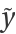is an approximate solution to this ODE, we can define its error as the absolute value of the remainder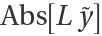.
ResourceFunction["FrobeniusPiecewiseDSolve"] places the piecewise subintervals such that the error (as defined above) of the approximate solutions it outputs does not exceed some fixed margin given by the option Tolerance.
Frobenius series solutions are quick to compute and very accurate; however, they generally only have a finite convergence radius, which limits their applications. This issue can be overcome with the introduction of the piecewise Frobenius method, which solves ODEs by "gluing" several series solutions together under the condition of continuity.
Read more about the working principle of the function in the related Wolfram Community post.

## Examples

### Basic Examples (3)

Obtain an approximate piecewise Frobenius solution to a differential equation:

 In:=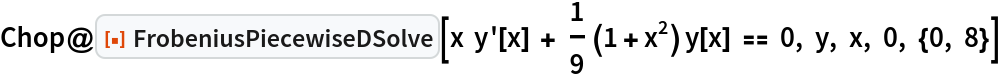Out=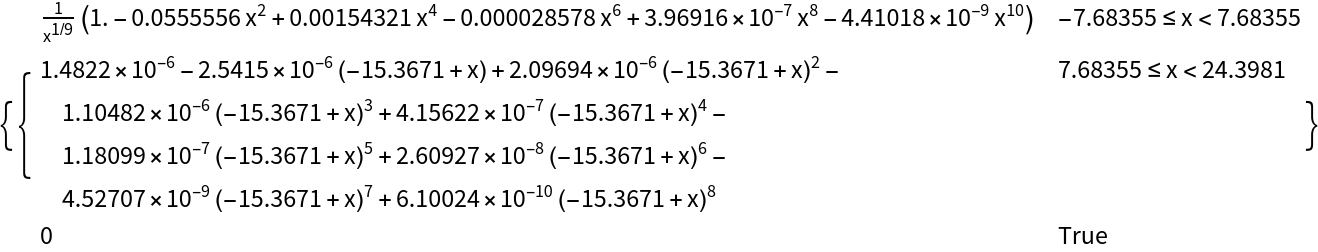Compute an approximate solution to the ODE 2x2(x+3)2y''+7x(x+3)y'-3y=0 in the domain x[0,6] using piecewise Frobenius method:

 In:=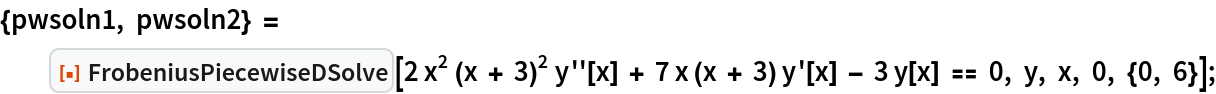Each of the two solutions is a piecewise Frobenius series. Display the two solutions, omitting for shortness fourth and higher order terms:

 In:=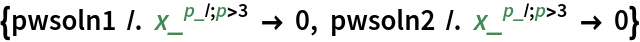Out=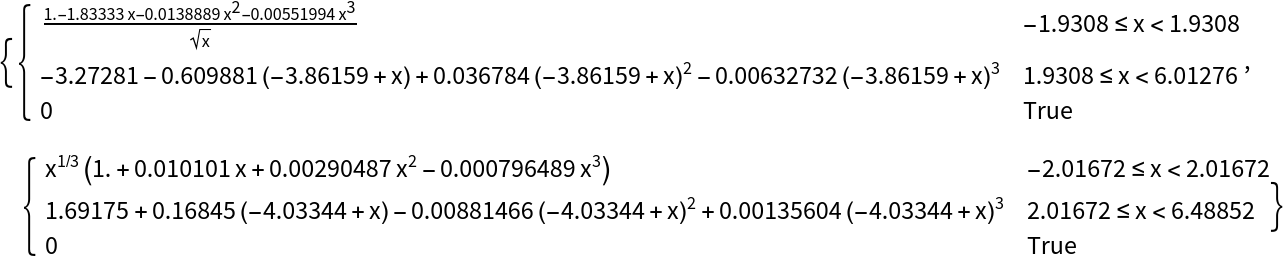By default, the maximum power of x in each of the Frobenius series is 28 (see Options). Plot the solutions:

 In:=Out=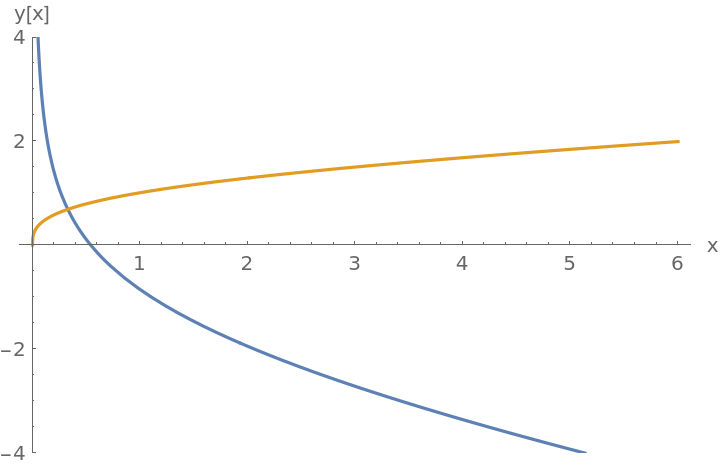Solve the non-singular ODE y''+(1+x2)y=0 for x between -8 and 8:

 In:=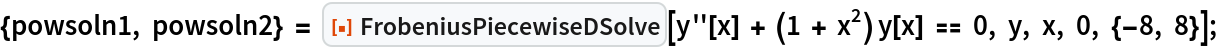Each solution is now a piecewise power series. Display the first solution up to order three:

 In:=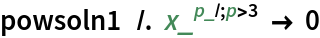Out=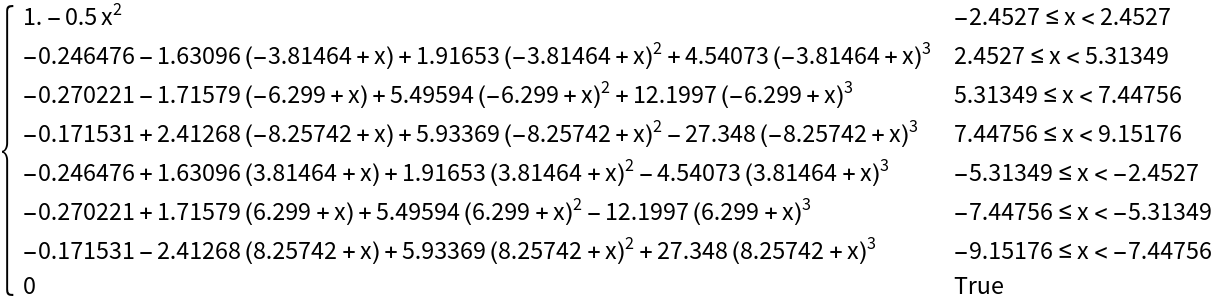Solutions to the equation y''+(1+x2)y=0 start oscillating really fast for large x, and to maintain the requested accuracy, FrobeniusPiecewiseDSolve uses many subintervals. Plot the solutions:

 In:=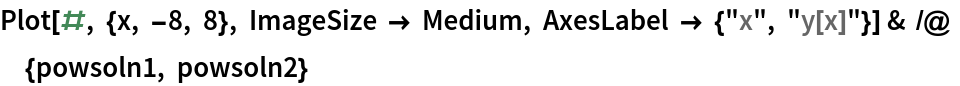Out=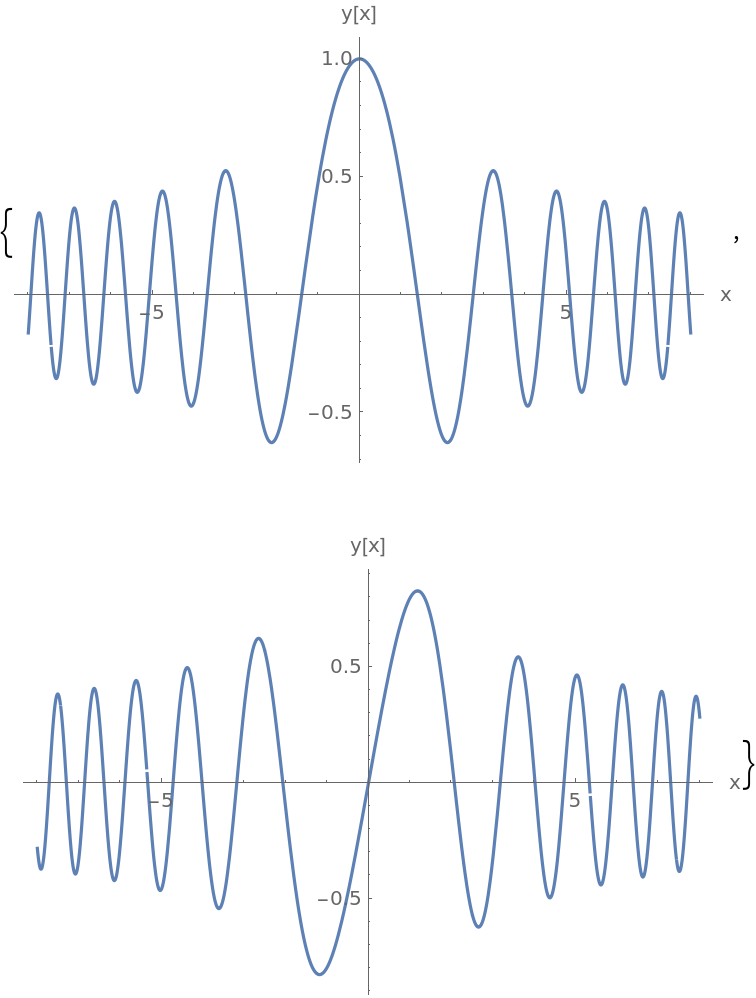### Scope (2)

The function can operate with complex numbers:

 In:=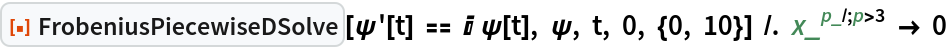Out=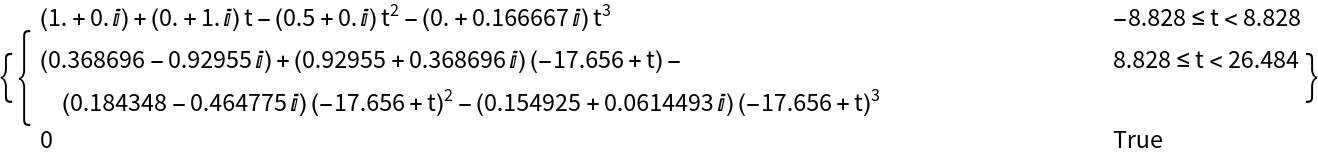FrobeniusPiecewiseDSolve can be used to solve ODEs of any order. Here, for example, are four linearly independent solutions to y''''[t]+ty[t]=0, with fourth and higher order terms omitted for shortness:

 In:=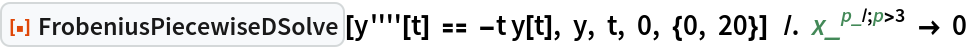Out=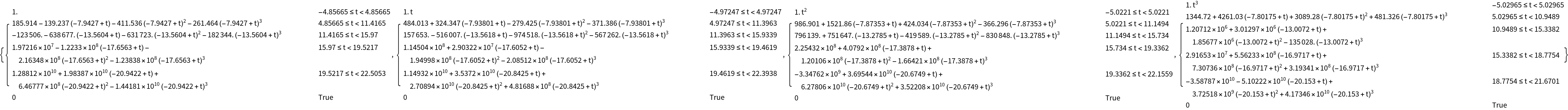### Options (2)

The option "FrobeniusOrder" controls the number of terms in each of the Frobenius series making up the piecewise solution:

 In:=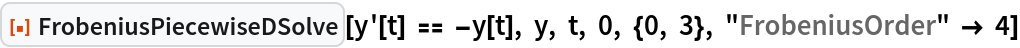Out=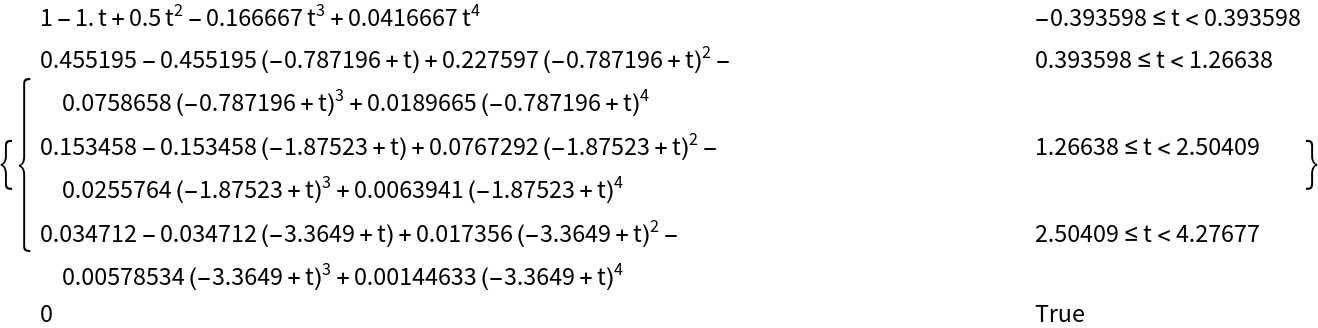FrobeniusPiecewiseDSolve can output solutions of any specified accuracy using the Tolerance option:

 In:=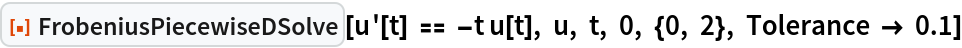Out=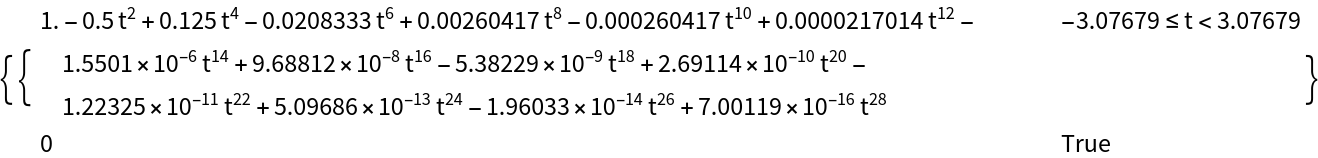The default value of Tolerance is 10-3. Compute the two linearly independent solutions to 2x2(x+3)2y''+7x(x+3)y'-3y=0 with Tolerance set to 10-12, and then plot the remainder of one of the solutions:

 In:=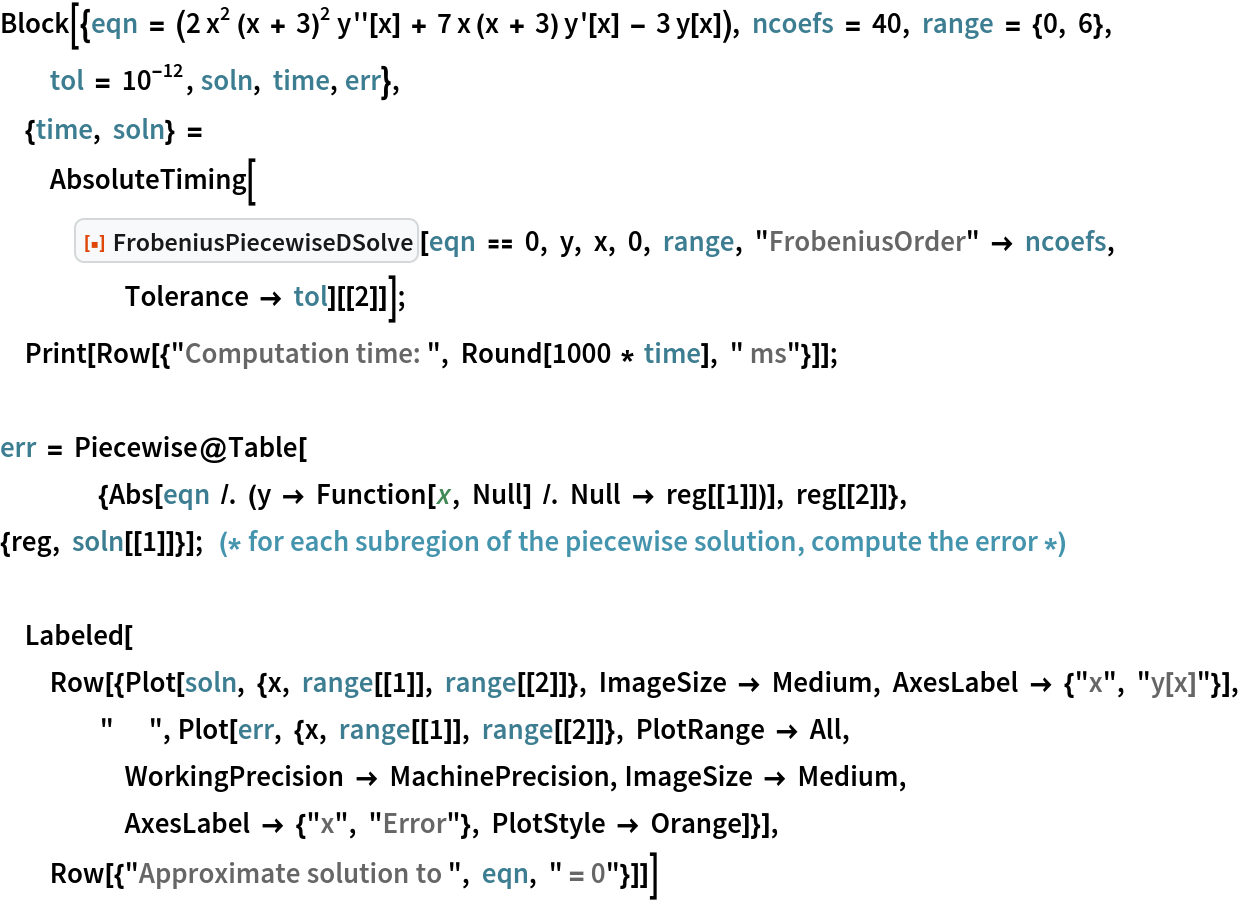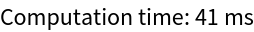Out=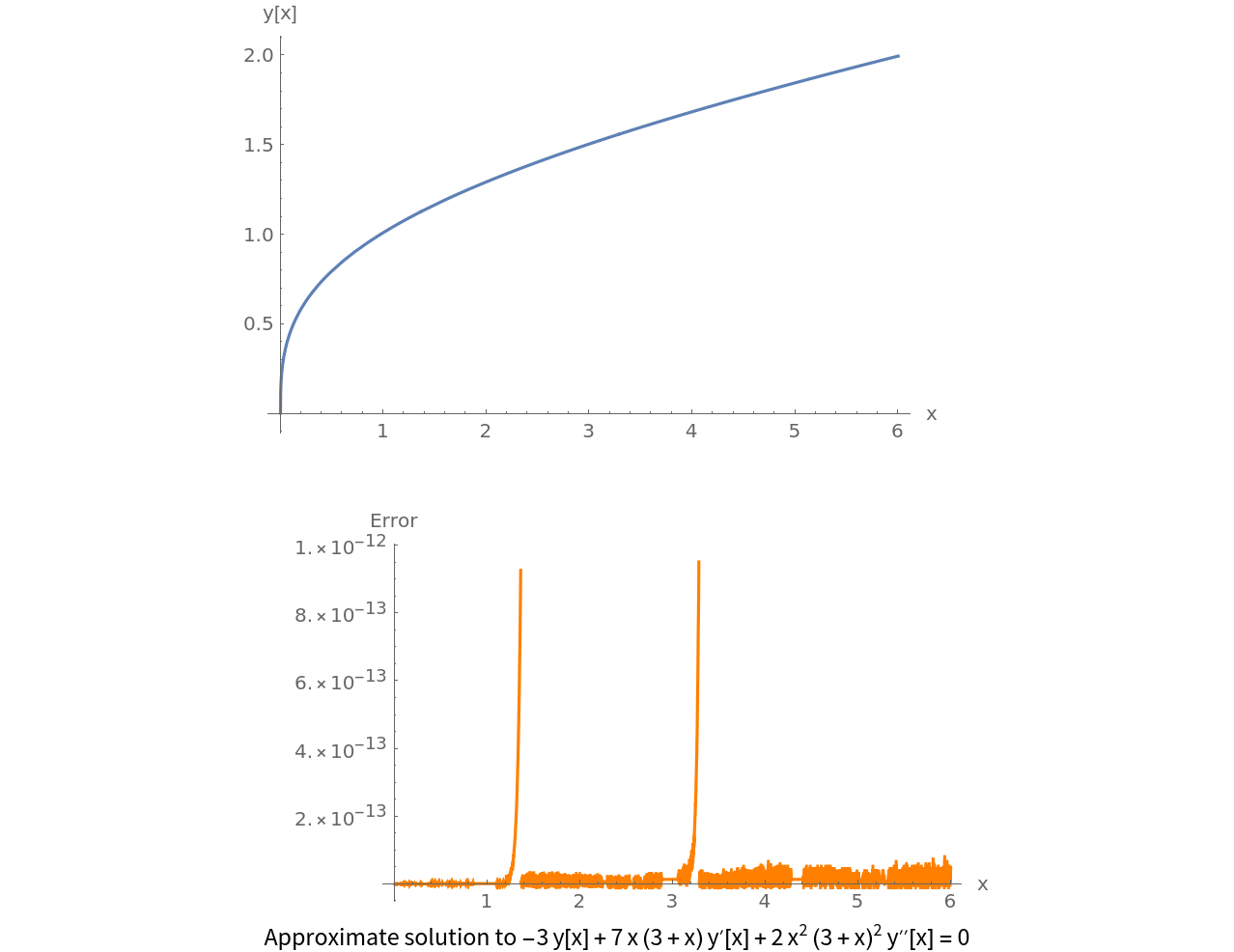As you can see, error stays below the tolerance of 10-12. This shows that FrobeniusPiecewiseDSolve can be used to quickly compute extremely accurate solutions. Here, the error of an approximate solution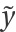to an ODE of the form L y = 0 is defined to be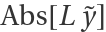, where L is a linear operator. For the ODE above, the error is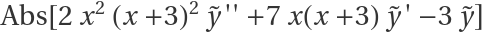.

### Properties and Relations (1)

The time it takes FrobeniusPiecewiseDSolve to compute a solution can be significantly reduced with the help of FrobeniusPiecewiseDSolveFormula:

 In:=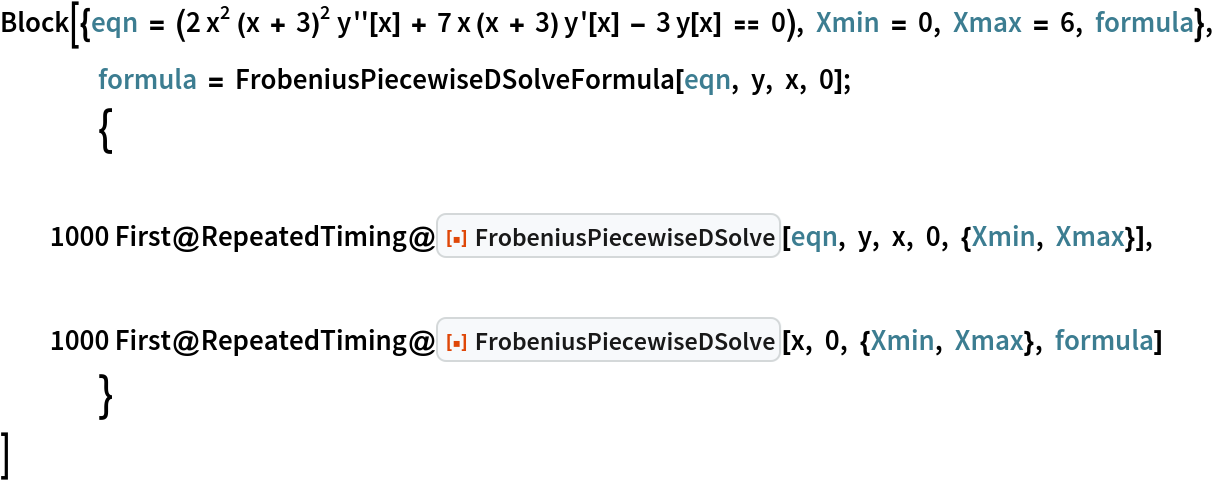Out=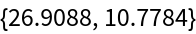FrobeniusPiecewiseDSolve first computes a formula and then uses it to calculate the solution. FrobeniusPiecewiseDSolveFormula allows us to compute the formula separately, which can help significantly reduce computation time when an ODE is to be solved repeatedly.

### Possible Issues (2)

The issues of underflow and overflow may arise if the option "FrobeniusOrder" is made too large. Solve the ODE 2x2(x+3)2y''+7x(x+3)y'-3y=0 with "FrobeniusOrder" set to 200, way above the default value of 28:

 In:=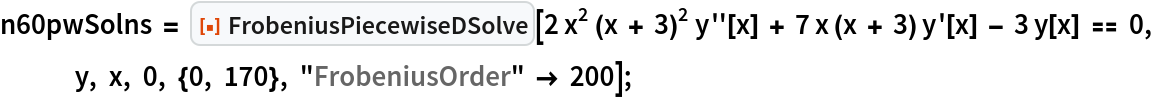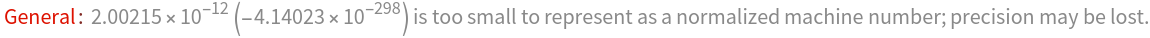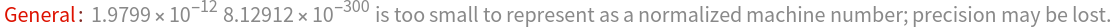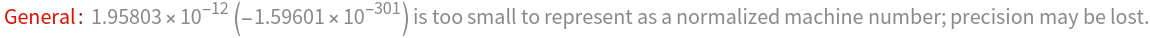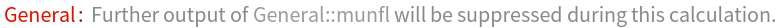As "FrobeniusOrder" gets larger, the function has to compute coefficients aj with larger and larger indexes. And because these coefficients typically decrease with index, at some point the value of the coefficients gets too small for the kernel to process. This makes FrobeniusPiecewiseDSolve underestimate the error of the series solutions, and as a result, it is unable to properly place the subintervals, resulting in an exploding solution:

 In:=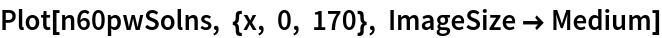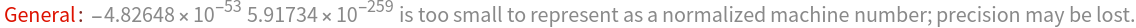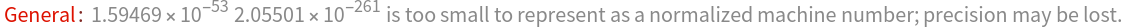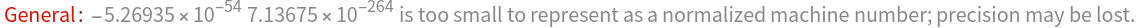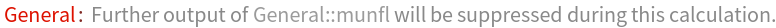Out=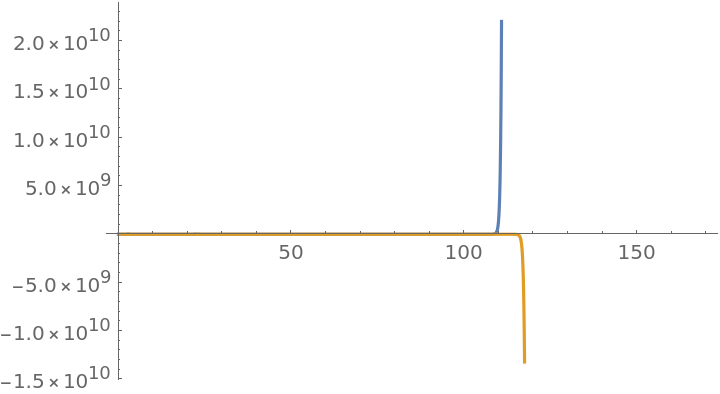When solving a given ODE in the region axb, FrobeniusPiecewiseDSolve assumes that the ODE either has a singular point at x=a, at x=b, or nowhere between a and b. The algorithm was built on the assumption of continuity. If there is a singular point between a and b, the function will enter an infinite cycle trying to get through the singular point:

 In:=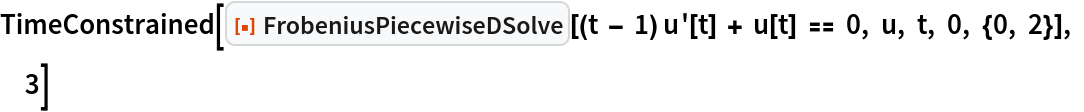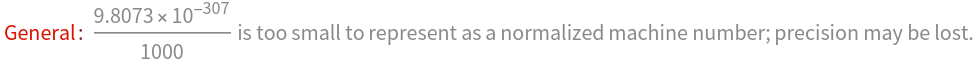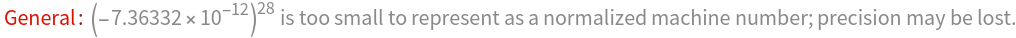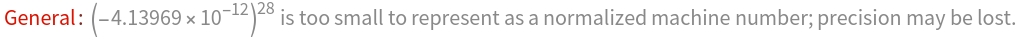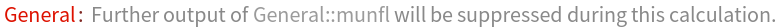Out=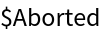This is not a big problem though, as the ODEs we solve on practice either don't have singular points, or the singularities are located in such a way that we wouldn't need to pass through them.

## Publisher

Wolfram Summer Camp

## Version History

• 1.1.0 – 19 April 2021